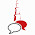# Types of Variables in java

In this post, we will talk about java variables, Types of Variables in java with complete explanation and examples. Like a different type of variable how to use it?. how to access it etc?. So java is the class base language the class contains five elements.

### Java Class Elements are

1. Variable
2. Methods
3. Constructor
4. Instance block
5. Static block

### 1. Variables in Java

So C/C++ language contains variable int a=10 b=20 etc. in the same way java also contains some variable part. so to work with employe project we need employee id, name, salary etc. So every project required variable concepts. So before going to the variable concept. First of all, we must know what is the purpose of the variable in java. So the variable is used to store the values by using these value we achieve the project requirements. For example, an employee project, we need employee salary, name, id etc.

So in a single line variable are used to store value and by using these value we achieve the project requirements. generally, variables are 3 types.### Types of Variable

• Local Variables
• Instance Variables
• Static Variables

### 1. Local Variables

1. The variable which is declared inside method or constructor or blocks also. Those variables called local variables

Example:-

class
{
public static void main(String[] args)
{
//local variables
int a=50;
int b=20
System.out.println(a);
System.out.println(a);
}
}

2. The scope of the local variables only within the method only or within the constructor or within the blocks only. So simply can say that local variable cannot be accessed outside the method, block, constructors. If we trying to access the local variable outside it generates the error message.

Example:-

class demo
{
public static void main(String[] args)
{
void method()
{
int a=30;
System.out.println(a); //possible
}
void method2()
{
System.out.println(a);// not possible to print inside the method
}
}
}

3. local variable Memory Allocated when method start and the whenever the method is completed memory is released. Local variable stored in stack memory.

### 2. Instance Variables

So before the brief of instance variables, we must know areas of java language.java contains 2 types of areas, instance area, and static area.so once we understand these 2 areas then java variables concept is very easy. So the body of instance method called instance area, and the body of the static method called the static method.

Example:-

class demo
{
void method()// instance method
{
//instance area
}
static void method2() //static method
{
//static area
}
public static void main(String[] args)
{
}
}

1. The variable that is declared inside the class but outside the method, Those variables called instance variables.

2. The scope of the instance variable is inside the class all methods, constructor and blocks are able to access.

3. For the instance variable memory allocated when the object is created and memory is destroyed when the object is destroyed.

4. Instance variable stored in heap memory

Note:- When the same area we can access the instance variable directly. But in a different area, first of all, we must allocate memory of instance variable So how memory is allocated, linked with the object, means create an object of that class in a single line, first of all, create an object of that class. And access that class instance variable and method by using that object.

Instance var, method==========>direct access========>instance area

Instance var,method==============>throw object========>static area

Example:-

class demo
{
//instance var
int x=20;
int y=30;
void m()//instance method
{
//instance area
System.out.println(x+y);
}

public static void main(String[] args)//main method (static method)
{
//static area
demo d new demo();
System.out.println(d.x);
System.out.println(d.y);
d.m();
}
}

### 3. Static Variable

1. The variable that is declared inside the class but outside the method with a static modifier, Those variables called static variables.

Example:-

class
{
//static variables
static int a;
static float b;
static char c;
}

2. Static variable memory allocated when the .class file is loading.
3. The scope of the static variable is inside the class
4. Static variable stored in non-heap memory.

Note:- access the static variable and method by using the class name. Reason static variable memory allocated when the .class file is loading. means before the main method load, static variable memory allocated.

Example:-

class demo
{
//static var
static int x=20;
static int y=30;
void m()//instance method
{
//instance area
System.out.println(demo.x);
System.out.println(demo.y);
}
public static void main(String[] args)//main method (static method)
{
//static area
demo d = new demo();
System.out.println(demo.x);
System.out.println(demo.y);
d.m();
}

1.2.